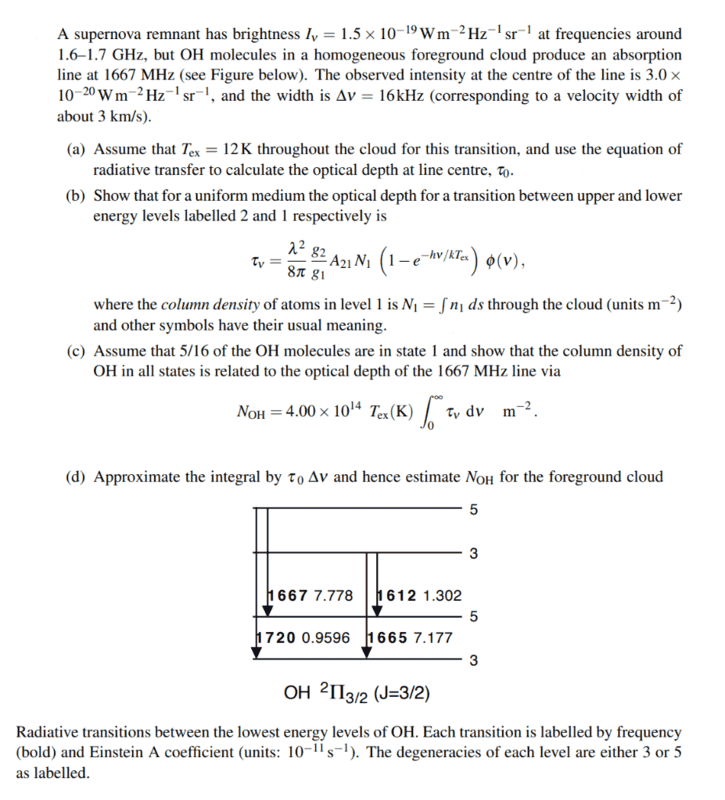# Optical depth from radiative transfer equation

• Kayla Martin
Kayla Martin
Homework Statement
Hi, I can't figure out how to find the optical depth for the following situation:
A supernova remnant has a brightness of I=1.5x10^{-19} Wm^{-2}Hz^{-1}sr^{-1} at frequencies around 1.6-1.7 GHz, but OH molecules in a homogenous foreground cloud produce an absorption line at 1667MHz. The observed intensity at the center of the line is 3.0x10^{-20} Wm^{-2}Hz^{-1}sr^{-1} and the width is 16kHz (corresponding to a velocity width of about 3km/s).
Assuming T_{ex}=12K throughout the cloud for this transition I need to use the radiative transfer equation to calculate the optical depth at the center of the line, tau_0.
Can someone please help me figure this out? I know we have been given all the equations for this in our lecture notes, but I am stumped at how to put it all together?
Relevant Equations
$$\frac{dI}{dS} = - \alpha I + j$$ where $$\alpha$$ is absorption coefficient...
$$\frac{dI}{\tau} = - I + S$$ where S is the source function $$S = \frac{j}{\alpha}$$ and $$\tau$$ is the optical depth.Last edited:

The only solution (for part a) I can think of is if we use the radiative transfer equation without S... i.e. $$\frac{I}{\tau}= I_0 e^{-\tau}$$ and then take the natural log of each side to get $$\tau= -\ln(\frac{I}{I_0})$$ but I don't know if I can just get rid of S like that?